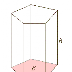Prism

A prism is a polyhedron with two parallel and congruent polygonal bases, All lateral faces (or sides) are parallelograms. Lateral faces meet in line segments called lateral edges.

A right prism is one whose lateral faces and lateral edges are perpendicular to its bases. The lateral faces of a right prism are all rectangles, and the height of a right prism is equal to the length of its lateral edge. A regular prism has regular polygons as bases and has all sides equal in length and all angles equal in measure. A right regular prism is one with regular polygon bases and perpendicular rectangular lateral sides.

The volume of a prism is: B * hThe Lateral Surface is: P*h The Surface of prism is: 2*B + Lateral surface area

where B is the area of base and P is the perimeter of the base

A regular right triangular prism is made of two equilateral Triangular bases and three identical rectangular sides:

|| Pyramid ||

Online Coaching (Use Coupon Code "QMATHS" to get 25-40% Discount)

PrismReviewed by Admin on 5:09:00 PM Rating: 5